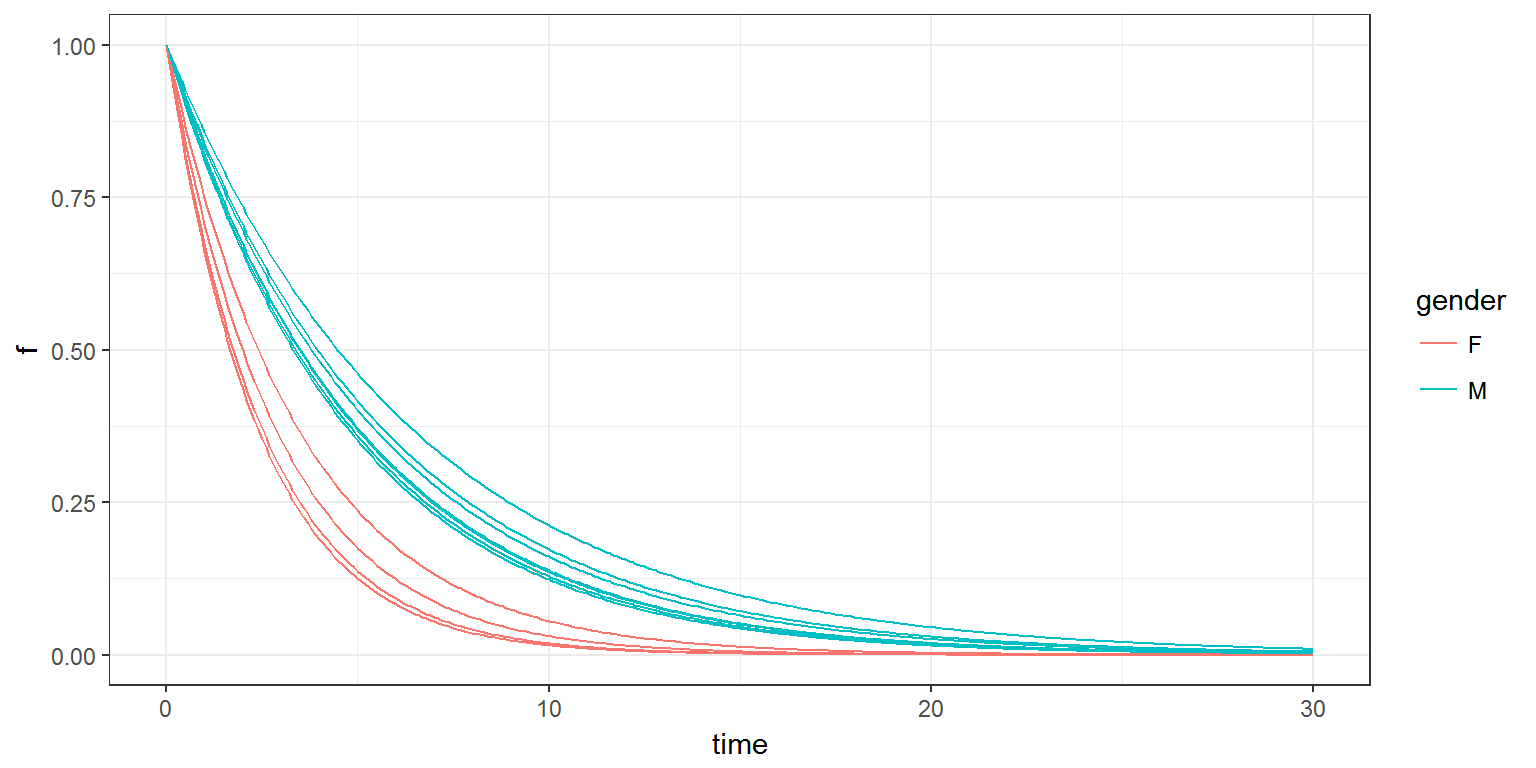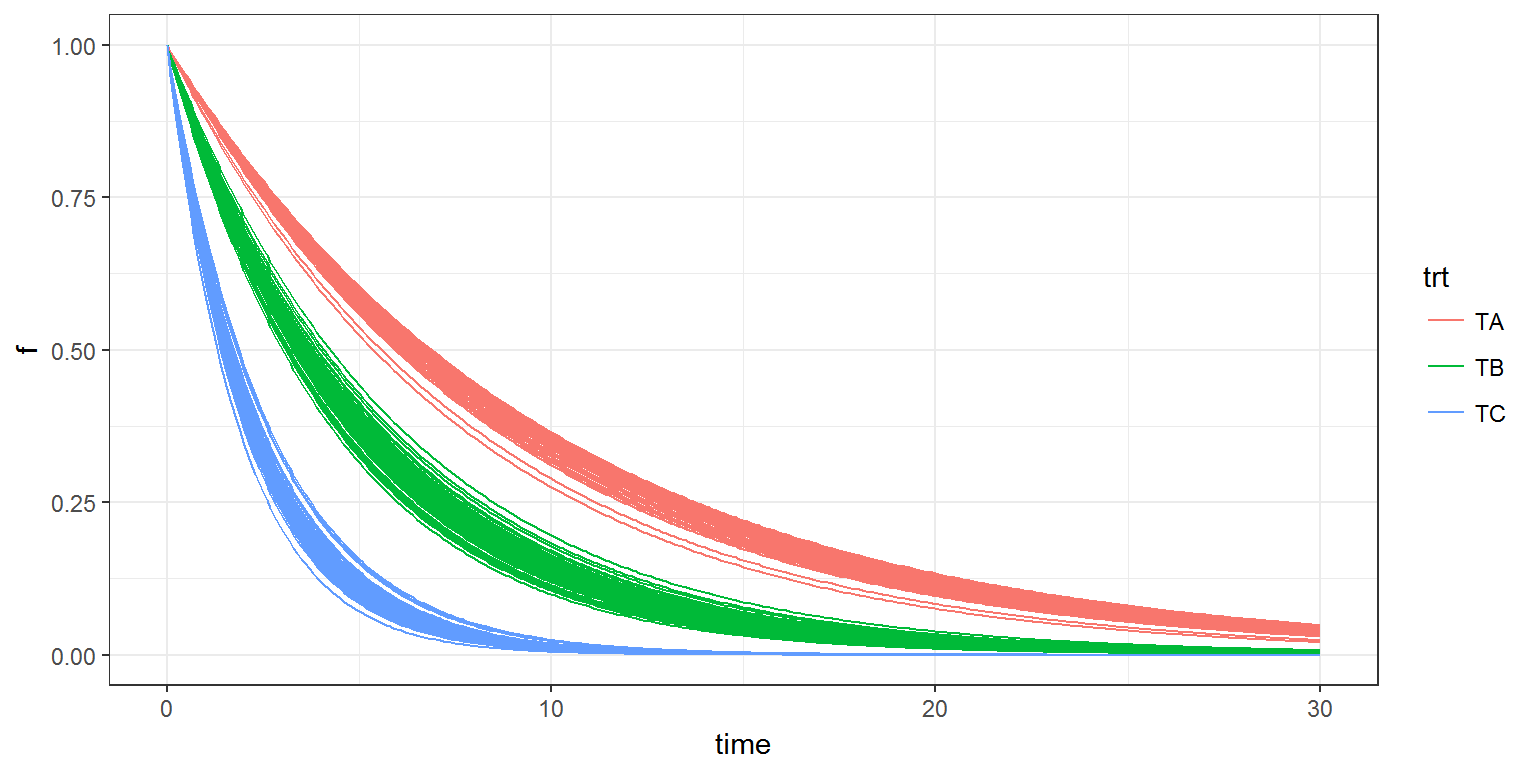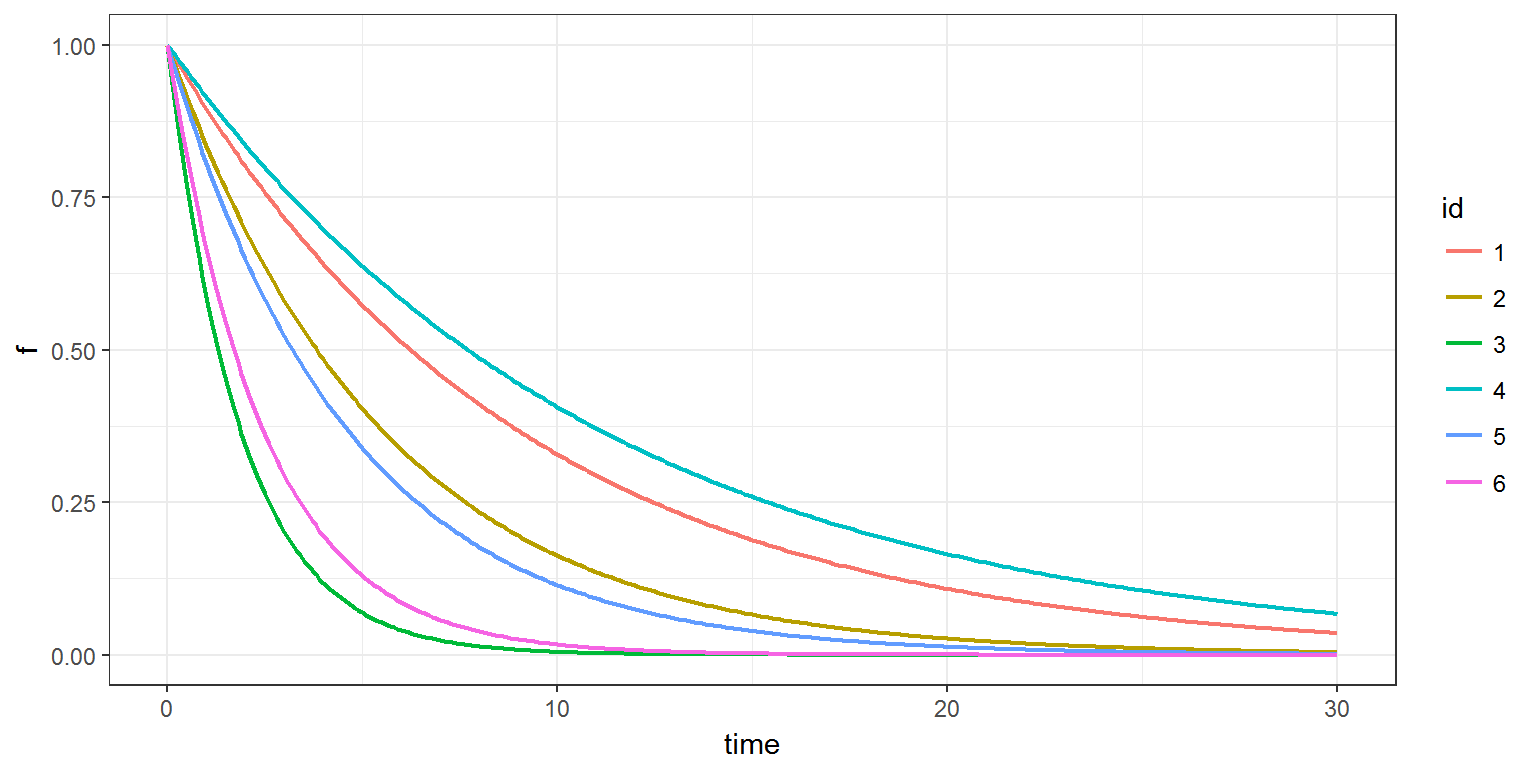R scripts: catcov.R

Mlxtran codes: model/catcov1A.txt ; catcov1B.txt ; catcov2A.txt ; catcov2B.txt

$$\newcommand{\esp}{\mathbb{E}\left(#1\right)} \newcommand{\var}{\mbox{Var}\left(#1\right)} \newcommand{\deriv}{\dot{#1}(t)} \newcommand{\prob}{ \mathbb{P}\!(#1)} \newcommand{\eqdef}{\mathop{=}\limits^{\mathrm{def}}} \newcommand{\by}{\boldsymbol{y}} \newcommand{\bc}{\boldsymbol{c}} \newcommand{\bpsi}{\boldsymbol{\psi}} \def\pmacro{\texttt{p}} \def\like{{\cal L}} \def\llike{{\cal LL}} \def\logit{{\rm logit}} \def\probit{{\rm probit}} \def\one{{\rm 1\!I}} \def\iid{\mathop{\sim}_{\rm i.i.d.}} \def\simh0{\mathop{\sim}_{H_0}} \def\df{\texttt{df}} \def\res{e} \def\xomega{x} \newcommand{\argmin}{{\rm arg}\min_{#1}} \newcommand{\argmax}{{\rm arg}\max_{#1}} \newcommand{\Rset}{\mbox{\mathbb{R}}} \def\param{\theta} \def\setparam{\Theta} \def\xnew{x_{\rm new}} \def\fnew{f_{\rm new}} \def\ynew{y_{\rm new}} \def\nnew{n_{\rm new}} \def\enew{e_{\rm new}} \def\Xnew{X_{\rm new}} \def\hfnew{\widehat{\fnew}} \def\degree{m} \def\nbeta{d} \newcommand{\limite}{\mathop{\longrightarrow}\limits_{#1}} \def\ka{k{a}} \def\ska{k{\scriptscriptstyle a}} \def\kel{k{e}} \def\skel{k{\scriptscriptstyle e}} \def\cl{C{\small l}} \def\Tlag{T\hspace{-0.1em}{lag}} \def\sTlag{T\hspace{-0.07em}{\scriptscriptstyle lag}} \def\Tk{T\hspace{-0.1em}{k0}} \def\sTk{T\hspace{-0.07em}{\scriptscriptstyle k0}} \def\thalf{t{1/2}} \newcommand{\Dphi}{\partial_\pphi #1} \def\asigma{a} \def\pphi{\psi} \newcommand{\stheta}{{\theta^\star}} \newcommand{\htheta}{{\widehat{\theta}}}$$

# 1 Introduction

Categorical variables take a finite number of values from a set that is not necessarily numerical or even ordered, e.g., gender, country or ethnicity.

The approach taken for continuous covariates extends easily to categorical ones. For simplicity’s sake, let us consider a unique covariate $${c}_i$$ that takes its values in $$\{ a_1, a_2, \ldots, a_K\}$$, and a unique parameter $$\psi_i$$.

Here, a reference covariate value $${c}_{\rm pop}$$ is a reference category, i.e., a specific element $$a_{k^\ast}$$ of $$\{ a_1, a_2, \ldots, a_K\}$$.

Assume for instance a linear model of covariates. Then, the prediction $$\tilde{\psi}_i$$ for $$\psi_i$$ is given by :

$h(\tilde{\psi}_i) = h(\psi_{\rm pop})+ \beta_1 \one_{{c}_i=a_1} + \beta_2 \one_{{c}_i=a_2} + \ldots + \beta_K \one_{{c}_i=a_K} ,$

with $$\beta_{k^\ast} = 0$$ and where $$\one$$ is the indicator function ($$\one_A = 1$$ if $$A$$ is true, 0 otherwise).

This model is equivalent to $h(\tilde{\psi}_i) = \left\{ \begin{array}{ll} h(\psi_{\rm pop}) & \hbox{if {c}_i=a_{k^\ast}} \\ h(\psi_{\rm pop}) + \beta_k & \hbox{if {c}_i=a_k \neq a_{k^\ast}} . \end{array} \right.$

We see that if the covariate has $$K$$ categories, $$K-1$$ coefficients $$(\beta_k)$$ are required for defining the covariate model.

# 2 Example 1

Consider a model where gender takes the values $$M$$ and $$F$$ with $\prob{{\rm gender}_i=F} = p_F$

Then, gender is used as a categorical covariate for describing the variability of $$k$$

$\log(k_i) = \log(k_{\rm pop}) + \beta_F \one_{{\rm gender}_i=F} + \eta_i$

That means that the typical values of $$k$$ for a male and a female are, respectively, $$k_{\rm pop}$$ and $$k_{\rm pop}e^{\beta_F}$$.

Function of time is then defined as $f(t ; k_i) = e^{-k_i \, t}$

This model is implemented in catcov1A.txt:

[COVARIATE]
input={p_F}

DEFINITION:
gender = { type        = categorical,
categories  = {F,M},
P(gender=F) = p_F }

;---------------------------------------
[INDIVIDUAL]
input={k_pop, omega_k, gender, beta_F}
gender={type=categorical,categories={F,M}}

DEFINITION:
k = { distribution = lognormal,
reference    = k_pop,
covariate    = gender,
coefficient  = {beta_F,0},
sd           = omega_k }

;---------------------------------------
[LONGITUDINAL]
input =  {k}

EQUATION:
f = exp(-k*t)


Let us generate 12 simulated genders, 12 individual parameters $$k_i$$ (one per simulated gender) and 12 functions $$f(t;k_i)$$ (one per individual parameter) with this model:

p <- c(p_F=0.4,k_pop=0.2, omega_k=0.1, beta_F=0.6)
f <- list(name='f', time=seq(0, 30, by=0.1))
ind <- list(name=c("gender","k"))

res1 <- simulx(model    = "model/catcov1A.txt",
parameter= p,
output   = list(ind, f),
group    = list(size=12, level='covariate'),
settings = list(seed=12345))

print(head(res1$parameter)) ## id gender k ## 1 1 F 0.3967964 ## 2 2 M 0.1993954 ## 3 3 F 0.3479398 ## 4 4 M 0.2091361 ## 5 5 F 0.2885420 ## 6 6 M 0.1754243 fg <- merge(res1$f,res1parameter) plot(ggplot(data=fg) + geom_line(aes(x=time, y=f, group=id, colour=gender), size=0.5))Remark: simulx supports any alphanumeric (alphabetic and numeric) character to define the categories of a categorical variable in a block a block EQUATION or in a bloc DEFINITION. The model for $$k_i$$ can be represented in an equivalent manner as follows: \begin{align} \tilde{k}_i & = \left\{ \begin{array}{ll} k_{\rm pop} & \text{if } \ \ {\rm gender}_i=M \\ k_{\rm pop} e^{\beta_F} & \text{if } \ \ {\rm gender}_i=F . \end{array} \right. \\ \log(k_i) &= \log(\tilde{k}_i)) + \eta_i \end{align} This model is implemented in catcov1B.txt: [COVARIATE] input={p_F} DEFINITION: gender = { type=categorical, categories={F,M}, P(gender=F)=p_F } [INDIVIDUAL] input={k_pop, omega_k, gender, beta_F} gender={type=categorical,categories={F,M}} EQUATION: if gender==F k_pred = k_pop*exp(beta_F) else k_pred = k_pop end DEFINITION: k = {distribution=logNormal, prediction=k_pred, sd=omega_k} [LONGITUDINAL] input = {k} EQUATION: f = exp(-k*t)  Implementations catcov1A.txt and catcov1B.txt are equivalent. # 3 Example 2 Let us consider now an extension of the previous model, with a continuous covariate $$w$$ and a categorical covariate $$trt$$ with three categories $$TA$$, $$TB$$ and $$TC$$. The reference category is $$B$$ in this example. This model is implemented in catcov2A.txt: [COVARIATE] input = {w_pop, omega_w, p_A, p_B} DEFINITION: w = { distribution=normal, mean=w_pop, sd=omega_w } trt = { type=categorical, categories={TA,TB,TC}, P(trt=TA)=p_A, P(trt=TB)=p_B } ;------------------------------------------- [INDIVIDUAL] input = {k_pop, omega_k, w, trt} trt = {type=categorical,categories={TA,TB,TC}} EQUATION: lw70 = log(w/70) DEFINITION: k = { distribution = lognormal, reference = k_pop, covariate = {lw70,trt}, coefficient = {0.2,{-0.6,0,0.8}}, sd = omega_k } ;------------------------------------------- [LONGITUDINAL] input = {k} EQUATION: f = exp(-k*t)  We will use simulx for generating 200 individuals with different simulated covariates: p <- c(w_pop=70, omega_w=15, p_A=0.2, p_B=0.4, k_pop=0.2, omega_k=0.05) f <- list(name='f', time=seq(0, 30, by=0.1)) ind <- list(name=c('w','k','trt')) res3 <- simulx(model = "model/catcov2A.txt", parameter = p, output = list(ind, f), group = list(size=200, level='covariate'), settings = list(seed=12345)) print(head(res3parameter))
##   id        w         k trt
## 1  1 71.23123 0.2094233  TB
## 2  2 82.76591 0.2065015  TB
## 3  3 69.54589 0.4343591  TC
## 4  4 63.05680 0.2002886  TB
## 5  5 76.70018 0.4033726  TC
## 6  6 34.97849 0.1630424  TB
fg<-merge(res3$f,res3$parameter)
plot(ggplot(data=fg) + geom_line(aes(x=time, y=f, group=id, colour=trt), size=0.5))Until now, the categorical covariates were simulated using section [COVARIATE]of the model Mlxtran. Categorical covariates can also be used as inputs of the model.

FOr example, model catcov2B.txt has only two sections [INDIVIDUAL] and [LONGITUDINAL]:

[INDIVIDUAL]
input = {k_pop, omega_k, w, trt}
trt   = {type=categorical, categories={TA,TB,TC}}
EQUATION:
lw70 = log(w/70)
DEFINITION:
k = { distribution = lognormal,
reference    = k_pop,
covariate    = {lw70,trt},
coefficient  = {0.2,{-0.6,0,0.8}},
sd           = omega_k }

[LONGITUDINAL]
input =  {k}
EQUATION:
f = exp(-k*t)


The values of the covariates $$w$$ and $$trt$$ for 6 individuals are defined in the following R script. They are then added to the list of input parameters.

p.indiv <- inlineDataFrame("
id   w    trt
1  80     TA
2  60     TB
3  85     TC
4  55     TA
5  90     TB
6  60     TC
")
p.pop <- c(k_pop=0.2, omega_k=0.1)

f <- list(name='f', time=seq(0, 30, by=0.1))
ind <- list(name=c('w','k','trt'))

res4 <- simulx(model     = "model/catcov2B.txt",
parameter = list(p.indiv, p.pop),
output    = list(ind, f),
settings  = list(seed=123))

print(res4$parameter) ## id w k trt ## 1 1 80 0.11119100 TA ## 2 2 60 0.18139348 TB ## 3 3 85 0.53408323 TC ## 4 4 55 0.08999034 TA ## 5 5 90 0.21648495 TB ## 6 6 60 0.40827956 TC plot(ggplot(data=res4$f, aes(x=time, y=f, colour=id)) + geom_line(size=0.75))